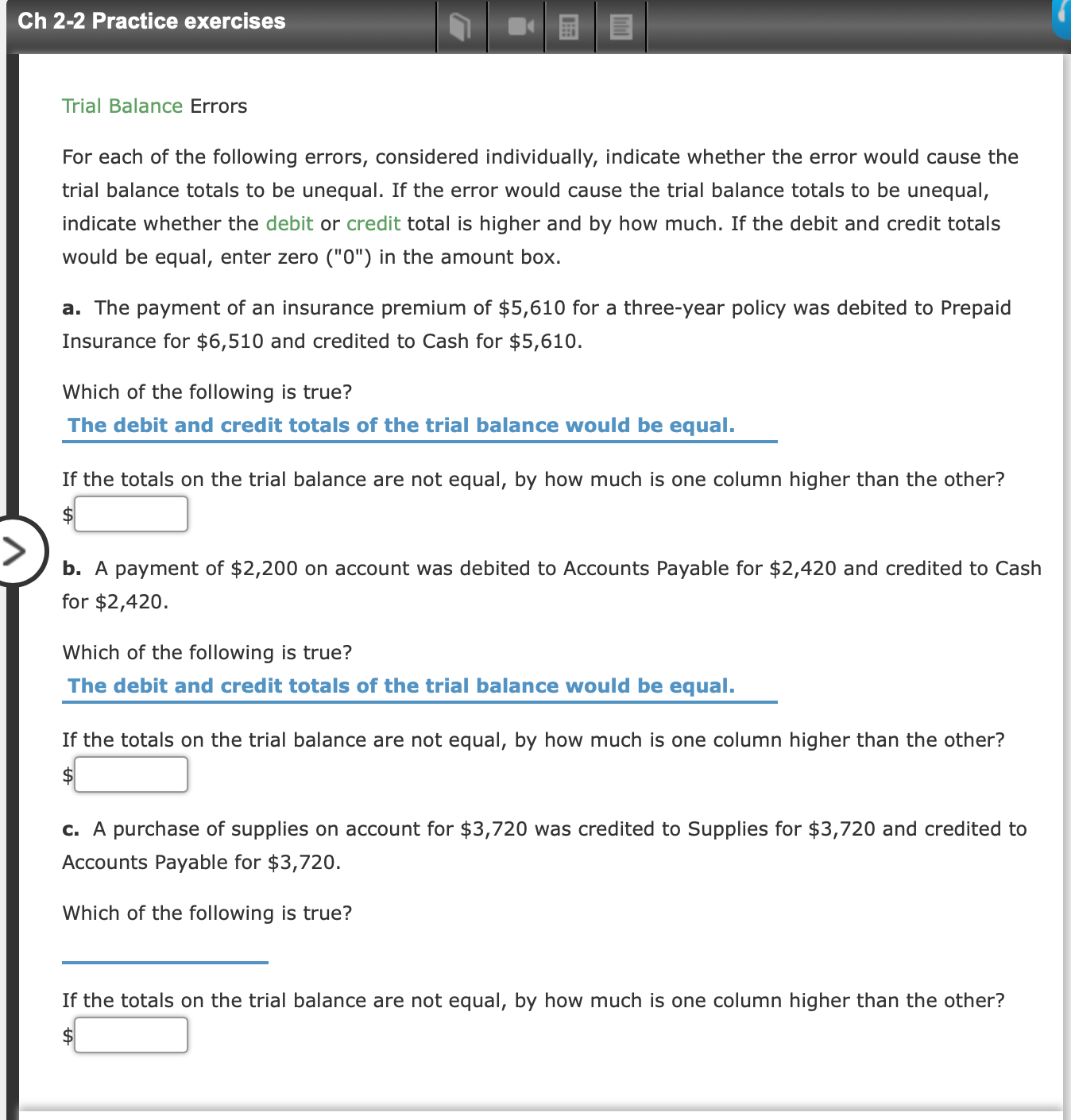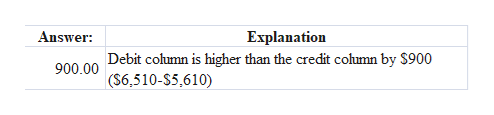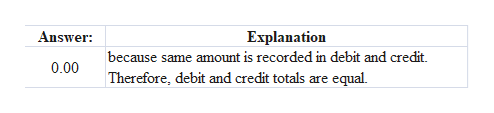Ch 2-2 Practice exercisesTrial Balance ErrorsFor each of the following errors, considered individually, indicate whether the error would cause thetrial balance totals to be unequal. If the error would cause the trial balance totals to be unequal,indicate whether the debit or credit total is higher and by how much. If the debit and credit totalswould be equal, enter zero ("0") in the amount boxa. The payment of an insurance premium of \$5,610 for a three-year policy was debited to PrepaidInsurance for \$6,510 and credited to Cash for \$5,610.Which of the following is true?The debit and credit totals of the trial balance would be equal.If the totals on the trial balance are not equal, by how much is one column higher than the other?b. A payment of \$2,200 on account was debited to Accounts Payable for \$2,420 and credited to Cashfor \$2,420Which of the following is true?The debit and credit totals of the trial balance would be equal.If the totals on the trial balance are not equal, by how much is one column higher than the other?\$c. A purchase of supplies on account for \$3,720 was credited to Supplies for \$3,720 and credited toAccounts Payable for \$3,720.Which of the following is true?If the totals on the trial balance are not equal, by how much is one column higher than the other?\$

Question

not sure how to slove those questionshelp_outlineImage TranscriptioncloseCh 2-2 Practice exercises Trial Balance Errors For each of the following errors, considered individually, indicate whether the error would cause the trial balance totals to be unequal. If the error would cause the trial balance totals to be unequal, indicate whether the debit or credit total is higher and by how much. If the debit and credit totals would be equal, enter zero ("0") in the amount box a. The payment of an insurance premium of \$5,610 for a three-year policy was debited to Prepaid Insurance for \$6,510 and credited to Cash for \$5,610. Which of the following is true? The debit and credit totals of the trial balance would be equal. If the totals on the trial balance are not equal, by how much is one column higher than the other? b. A payment of \$2,200 on account was debited to Accounts Payable for \$2,420 and credited to Cash for \$2,420 Which of the following is true? The debit and credit totals of the trial balance would be equal. If the totals on the trial balance are not equal, by how much is one column higher than the other? \$ c. A purchase of supplies on account for \$3,720 was credited to Supplies for \$3,720 and credited to Accounts Payable for \$3,720. Which of the following is true? If the totals on the trial balance are not equal, by how much is one column higher than the other? \$ fullscreen
Step 1

(a)help_outlineImage TranscriptioncloseAnswer Explanation 900.00 Debit column is higher than the credit column by \$900 (S6,510-S5,610) fullscreen
Step 2

(b)

...help_outlineImage TranscriptioncloseAnswer Explanation because same amount is recorded in debit and credit Therefore, debit and credit totals are equal 0.00 fullscreen

Want to see the full answer?

See Solution

Want to see this answer and more?

Our solutions are written by experts, many with advanced degrees, and available 24/7

See Solution
Tagged in